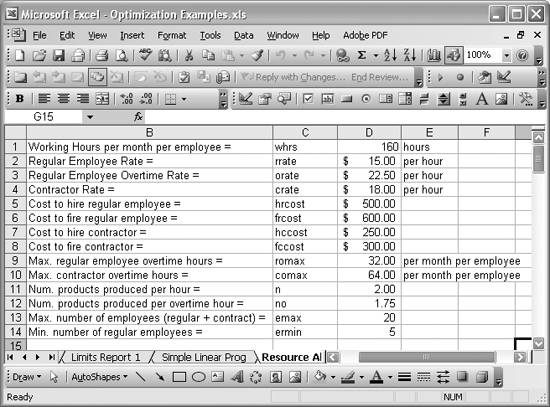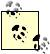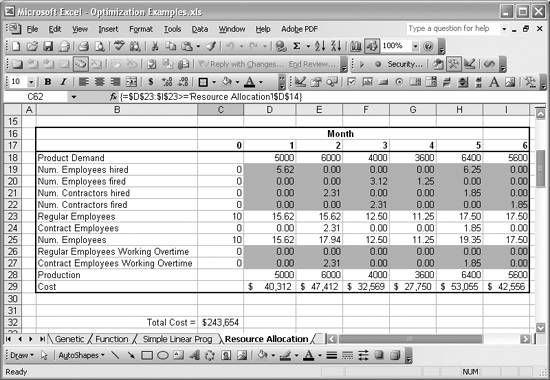# Exploring Resource Allocation Optimization Problems

Problem

You'd like to use Excel to solve a resource allocation problem.

Solution

You can use Excel and Solver to tackle more complicated linear optimization problems than what you saw in the previous recipe. Resource allocation problems, like finding the optimum product mix to yield maximum profit, are commonly formulated as linear optimization problems, which are easily solved in Excel. The following discussion shows you an example. The techniques discussed here are not limited to resource allocation problems ; on the contrary, they apply to many difficult problems so long as you can formulate them in the form of an objective function plus constraints.

Discussion

Let's assume you're running a laboratory that produces a certain chemical product. You currently employ 10 regular employees, technicians, who make the product. Given input from your sales and marketing group, you've put together a demand schedule for your product over the next six months. Operating budgets are tight, however, and you need to develop an optimum labor schedule for meeting demand for your product at minimum labor cost. I should point out that you know that the demand for your product over the next six months will fluctuate, so at some times you may need to hire new employees and at others you may have to let folks go.

You have the options of having your employees work overtime or hiring contract labor. Although they have a higher hourly rate than regular employees, contractors charge straight time for overtime, whereas you must pay regular employees time and a half for overtime.

Regular employees earn \$15 per hour (\$22.50 per overtime hour), while contract employees earn \$18 per hour (both regular and overtime hours). To complicate matters, regular employees can work only 8 hours overtime per week, due to union rules. Contract employees are willing to work up to 16 hours per week overtime.

Historically, you know that each employee is capable of producing 2 units of product per hour, but this productivity rate drops to about 1.75 units per overtime hour. Further, you have only enough space in your facility to employ a maximum of 20 technicians at any given time. Also, it is company policy to employ no less than 5 regular technicians at any given time.

Finally, your human resources director tells you that it costs \$500 in overhead charges to hire a regular employee and \$600 to let one go. Similarly, it costs only \$250 to hire a contractor and \$300 to let one go.

Your task now is to come up with a labor schedule for the next six months that will allow you to meet projected demand for your product, while at the same time minimizing labor costs.

This is a classic sort of linear optimization problem that's ideally suited for solving in Excel. I'll walk you through the process.

The first thing I did toward solving this problem was enter all the data in a spreadsheet. Figure 13-4 shows how I arranged this data.

The cells in column B contain text labels I used to describe the data. The actual data values are contained in column D. The short text labels you see in column C are labels I included to allow me to easily define cell names for each data item. I use these cell names in other cell formulas later.

Figure 13-4. Resource allocation problem dataTo quickly define names for a group of cells arranged like those shown in Figure 13-4, select the range of cells containing text label and data value pairs and then select Insert [Image_Link]/images/U2192.jpg border=0> Name [Image_Link]/images/U2192.jpg border=0> Create from the main menu bar. This will display a simple Create Names dialog box, allowing you to choose how you want the names created. You can choose to create the names given text labels in the left column, right column, or top or bottom row of the selected cell range. For this example, I selected the cell range C1:D14 and then selected Insert [Image_Link]/images/U2192.jpg border=0> Name [Image_Link]/images/U2192.jpg border=0> Create. Then I chose the Left Column option from the Create Names dialog box.

After setting up the data, I created a table in the spreadsheet to contain a monthly labor schedule. This part of the spreadsheet is shown in Figure 13-5.

The first row of this table contains the projected demand for your product over the next six months. These are given values. At month 0, the current month, you know you have 10 regular employees working regular 40-hour work weeks. The second to last row, Production, calculates the monthly production given the number of regular and contract employees and their overtime schedules each month. The formulas contained in this row look like this: =n*(D23+D24)*whrs+no*D26*romax+no*D27*comax. Clearly the projected production, given current staffing in month 0, is not going to be enough to meet the forecast demand. So your job is to figure out how many (if any) regular or contract employees to hire each month (along with their overtime schedules) to allow you to meet production targets at minimum labor cost.

Figure 13-5. Resource allocation monthly scheduleLabor cost, or Total Cost, is the objective function. In this case you want to minimize total cost. Cell C32 contains the total cost for this example. It is simply the sum of monthly costs computed in the last row of the table, the row labeled Cost.

Monthly cost is computed using formulas like =D23*whrs*rrate + D24*whrs*crate + D26*romax*orate + D27*comax*crate + D19*hrcost + D20*frcost + D21*hccost + D22*fccost. There are eight terms in this cost function:

D23*whrs*rrate

This term computes the direct labor cost per month, given the total number of direct employees in a given month, along with the number of regular working hours per month and the regular employee labor rate.

D24*whrs*crate

This term computes the contract labor cost per month, given the total number of contractors, along with their labor rate and the number of regular working hours per month.

D26*romax*orate

This term computes the overtime labor cost for regular employees, given the number of regular employees working overtime (assuming each employee works the maximum 8 hours per week overtime), along with the overtime labor rate.

D27*comax*crate

This term computes the overtime labor cost for contractors, given their rate and assuming that each contractor working overtime works the maximum 16 hours per week overtime.

D19*hrcost

This term computes the overhead cost of hiring regular employees, given the number of regular employees hired at the beginning of a month.

D20*frcost

The term computes the overhead cost of firing ("letting go" is a nicer way to say this, I suppose) regular employees, given the number of regular employees fired at the beginning of a month.

D21*hccost

This term computes the overhead cost of hiring contract employees, given the number of contract employees hired at the beginning of a month.

D22*fccost

The term computes the overhead cost of firing contract employees, given the number of contract employees fired at the beginning of a month.

Given this cost function, you can readily see the variables in this problem. They include the following: the number of regular employees hired, the number of regular employees let go, the number of contractors hired, the number of contractors let go, the number of regular employees working overtime, and the number of contractors working overtime each month. I highlighted all of the variable cells in the table shown in Figure 13-5 so you can readily see them.

The next step is to use Solver to optimize this problem. I basically told Solver to minimize the total cost in cell C32 by changing all the shaded cells in Figure 13-5, subject to a bunch of constraints. (See Recipe 9.4 for instructions on adding constraints in Solver.) The constraints for this problem are as follows:

Production meets or exceeds demand

The production values computed in cells D28 through I28 must be greater than the corresponding projected demand values contained in cells D18 through I18.

Greater than zero requirement

Each of the variables (shaded cells in Figure 13-5) must be greater than or equal to 0.

Maximum employees

There can be no more than 20 total employees in any given month.

Overtime consistency

The number of regular employees working overtime must be less than or equal to the total number of regular employees in a given month. Also, the number of contract employees working overtime must be less than or equal to the total number of contract employees in a given month.

Minimum regular employees

The total number of regular employees must be greater than the specified minimum number of regular employees in any given month.

Refer to the previous recipe and the introduction to Chapter 9 for details on setting up and using Solver. Running Solver for this example quickly yielded the results shown in Figure 13-6.

Figure 13-6. Resource allocation resultsAs you can see, Solver found a solution satisfying all the constraints. It turns out that the minimum labor costs is \$243,654, which is achieved through a combination of hiring and letting go both regular and contract employees over the six-month period. Some months will also require some contractor overtime. So far so good, but there's one problem: look at the required number of regular employee hires for month 1. Solver says you need 5.62 employees. How do you hire 62% of an employee? Well, either you hire some part-time labor, in which case you'd have to alter your model accordingly, or you require Solver to find an optimum solution using only integer values for the variables. This latter approach requires the use of integer constraints . In the next recipe I show you how to apply integer constraints to solve this same example problem.Excel Scientific and Engineering Cookbook (Cookbooks (OReilly))
ISBN: 0596008791
EAN: 2147483647
Year: N/A
Pages: 206
Authors: David M Bourg